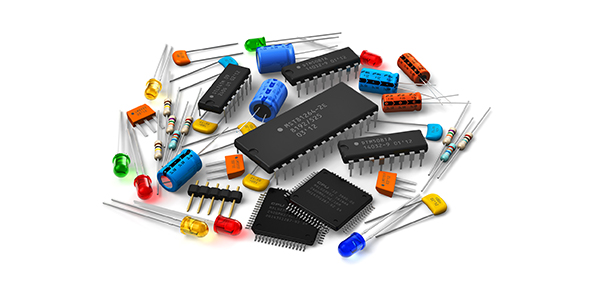# Gibilisco - Rlc And Glc Circuit Analysis

20 Questions | Total Attempts: 298SettingsCreate your own Quiz• 1.
Suppose a coil and capacitor are connected in series. The inductive reactance is 250 Ω, andthe capacitive reactance is −300 Ω. What is the complex impedance?
• A.

0 + j550

• B.

0 − j50

• C.

250 − j300

• D.

−300 + j250

• 2.
Suppose a coil of 25.0 µH and capacitor of 100 pF are connected in series. The frequency is5.00 MHz. What is the complex impedance?
• A.

0 + j467

• B.

25 + j100

• C.

0 − j467

• D.

25 − j100

• 3.
When R = 0 in a series RLC circuit, but the net reactance is not zero, the impedance vector
• A.

Always points straight up.

• B.

Always points straight down.

• C.

Always points straight toward the right.

• D.

None of the above is correct.

• 4.
Suppose a resistor of 150 Ω, a coil with a reactance of 100 Ω, and a capacitor with areactance of −200 Ω are connected in series. What is the complex impedance?
• A.

150 + j100

• B.

150 − j200

• C.

100 − j200

• D.

150 − j100

• 5.
Suppose a resistor of 330 Ω, a coil of 1.00 µH, and a capacitor of 200 pF are in series. Whatis the complex impedance at 10.0 MHz?
• A.

330 − j199

• B.

300 + j201

• C.

300 + j142

• D.

330 − j16.8

• 6.
Suppose a coil has an inductance of 3.00 µH and a resistance of 10.0 Ω in its winding. Acapacitor of 100 pF is in series with this coil. What is the complex impedance at 10.0 MHz?
• A.

10 + j3.00

• B.

10 + j29.2

• C.

10 − j97

• D.

10 + j348

• 7.
Suppose a coil has a reactance of 4.00 Ω. What is the complex admittance, assuming there is nothing else is in the circuit?
• A.

0 + j0.25

• B.

0 + j4.00

• C.

0 − j0.25

• D.

0 − j4.00

• 8.
What will happen to the susceptance of a capacitor if the frequency is doubled and all otherfactors remain constant?
• A.

It will decrease to half its former value.

• B.

It will not change.

• C.

It will double.

• D.

• 9.
Suppose a coil and capacitor are in parallel, with jBL =−j0.05 and jBC = j0.03. What is thecomplex admittance, assuming that nothing is in series or parallel with these components?
• A.

0 − j0.02

• B.

0 − j0.07

• C.

0 + j0.02

• D.

−0.05 + j0.03

• 10.
Imagine a coil, a resistor, and a capacitor connected in parallel. The resistance is 1.0 Ω, thecapacitive susceptance is 1.0 S, and the inductive susceptance is −1.0 S. Then, suddenly, thefrequency is cut to half its former value. What is the complex admittance at the new frequency?
• A.

1.0 + j0.0

• B.

1.0 + j1.5

• C.

1.0 − j1.5

• D.

1.0 − j2.0

• 11.
Suppose a coil of 3.50 µH and a capacitor of 47.0 pF are in parallel. The frequency is 9.55MHz. There is nothing else in series or parallel with these components. What is the complexadmittance?
• A.

0 + j0.00282

• B.

0 − j0.00194

• C.

0 + j0.00194

• D.

0 − j0.00758

• 12.
A vector pointing southeast in the GB plane would indicate
• A.

Pure conductance with zero susceptance.

• B.

Conductance and inductive susceptance.

• C.

Conductance and capacitive susceptance.

• D.

Pure susceptance with zero conductance.

• 13.
Suppose a resistor with conductance 0.0044 S, a capacitor with susceptance 0.035 S, and acoil with susceptance −0.011 S are all connected in parallel. What is the complex admittance?
• A.

0.0044 + j 0.024

• B.

0.035 − j0.011

• C.

−0.011 + j0.035

• D.

0.0044 + j0.046

• 14.
Suppose a resistor of 100 Ω, a coil of 4.50 µH, and a capacitor of 220 pF are in parallel.What is the complex admittance at a frequency of 6.50 MHz?
• A.

100 + j0.00354

• B.

0.010 + j0.00354

• C.

100 − j0.0144

• D.

0.010 + j0.0144

• 15.
Suppose the complex admittance of a circuit is 0.02 + j0.20. What is the complex impedance,assuming the frequency does not change?
• A.

50 + j5.0

• B.

0.495 − j4.95

• C.

50 − j5.0

• D.

0.495 + j4.95

• 16.
Suppose a resistor of 51.0 Ω, an inductor of 22.0 µH, and a capacitor of 150 pF are inparallel. The frequency is 1.00 MHz. What is the complex impedance?
• A.

51.0 − j14.9

• B.

51.0 + j14.9

• C.

46.2 − j14.9

• D.

46.2 + j14.9

• 17.
Suppose a series circuit has 99.0 Ω of resistance and 88.0 Ω of inductive reactance. An ac rms voltage of 117 V is applied to this series network. What is the current?
• A.

1.18 A

• B.

1.13 A

• C.

0.886 A

• D.

0.846 A

• 18.
What is the voltage across the reactance in the preceding example?
• A.

78.0 V

• B.

55.1 V

• C.

99.4 V

• D.

74.4 V

• 19.
Suppose a parallel circuit has 10 Ω of resistance and 15 Ω of reactance. An ac rms voltage of20 V is applied across it. What is the total current?
• A.

2.00 A

• B.

2.40 A

• C.

1.33 A

• D.

0.800 A

• 20.
What is the current through the resistance in the preceding example?
• A.

2.00 A

• B.

2.40 A

• C.

1.33 A

• D.

0.800 A

Related TopicsBack to top
×

Wait!
Here's an interesting quiz for you.